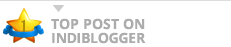# JavaScript Math Object | Methods, ExampleMath in JavaScript is a top-level, pre-defined object that has properties and methods for mathematical constants and functions. For example, Math object’s PI property contains the value of pi. The properties of Math object represent mathematical constants and its methods are mathematical functions. JavaScript Math object provides several constants and ... Read more The post JavaScript Math Object | Methods, Example appeared first on Scientech Easy.

blogs from Pune